Next: Calculating Fractal Dimension Up: Defining Fractals and Fractal Previous: Capacity Dimension

## Correlation Dimension

Correlation dimension can be calculated using the distances between each pair of points in the set of N number of points,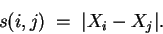A correlation function, C(r), is then calculated using,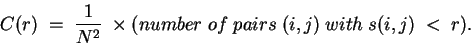C(r) has been found to follow a power law similar to the one seen in the capacity dimension: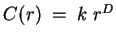. Therefore, we can find Dcorr with estimation techniques derived from the formula: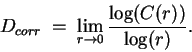C(r) can be written in a more mathematical form as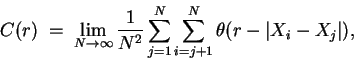where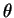is the Heaviside step function described as,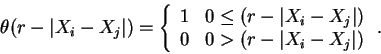Sandip Thanki
1999-07-29